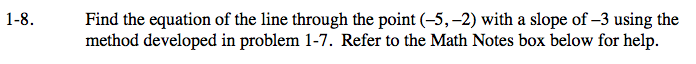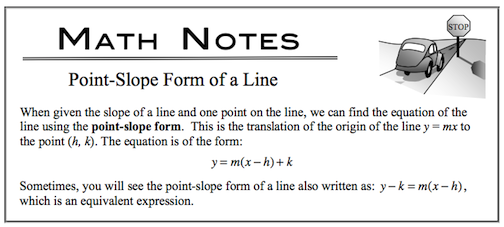### Home > CALC > Chapter 1 > Lesson 1.1.1 > Problem1-8

1-8.

Find the equation of the line through the point (−5, −2) with a slope of −3 using the method developed in problem 1-7. Refer to the Math Notes box below for help. Homework Help ✎Leave your answer in Point-Slope Form. There is no reason (in this case) to convert this equation into y-intercept form.